Gearing towards digital Education    0716 858 334

# Atomic Structure and the Periodic Table

Change Topic

### Guest Account

Attempt Form Two Chemistry Questions
Guest Account
Hello guest, kindly login to access unlimited study notes and revision questions.

Create an account / login to help track your progress and unlock more features including additional notes, rapid quizzes, revision questions, virtual library, and the new Esoma Classroom

# Structure of the Atom and the Periodic Table

## Simple Structure of the Atom

The are there are about 119 known elements
The following illustration shows the names and symbols of first 20 elements of the periodic table.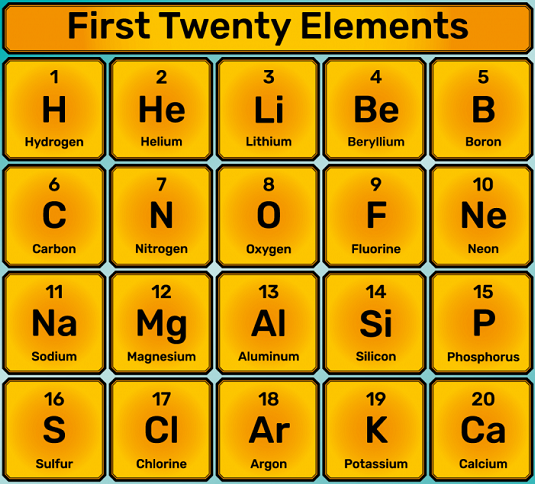Atoms are very small particles of an element, but they contain even smaller particles called sub-atomic particles as follows;
• Protons - found in the nucleus, they are positively charged (+ve)
• Electrons - found in the nucleus, they are negatively charged (–ve)
• Neutrons - they can be imagined as circulating the nucleus in energy levels, they have no charge
In a neutral atom, Number of protons = Number of electrons
Particles present in an atom are summarized below.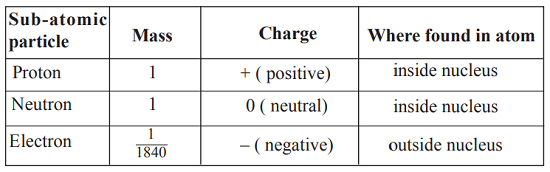### Structure of the Atom

The various energy levels in an atom are represented by a series of circles sharing the same centre (nucleus), separated from each other by roughly equal distances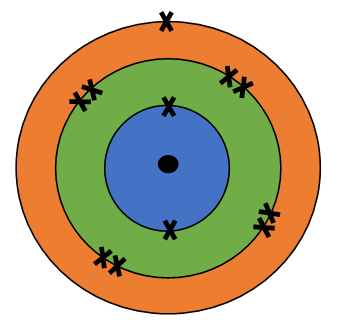The nucleus of the atom is at the centre of the circles. The electrons in the energy levels are represented by dots (.) or crosses (x). The energy levels are labelled 1st, 2nd, 3rd, 4th and so on starting from the one nearest to the nucleus as shown below;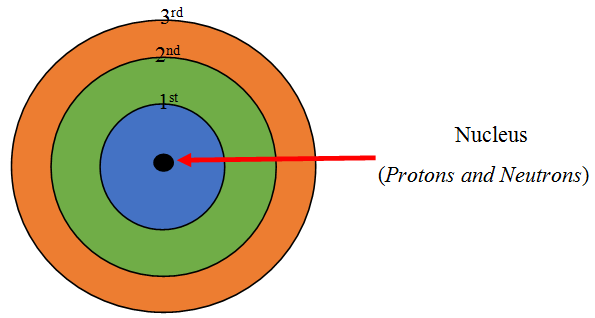The electrons that occupy the 1st energy level have lower energy than those in the 2nd energy level. Subsequently those in the 2nd energy level have lower energy than those in the 3rd energy level and so on. The 1st energy level usually has a maximum of two electrons while the 2nd energy level has a maximum of eight (8) electrons.

We can summarise the electrons, and their arrangement in each energy level as shown in the table below.
Element Symbol Electron
Configuration
Hydrogen H 1
Helium He 2
Lithium Li 2.1
Beryllium Be 2.2
Boron B 2.3
Carbon C 2.4
Nitrogen N 2.5
Oxygen O 2.6
Fluorine F 2.7
Neon Ne 2.8
Sodium Na 2.8.1
Magnesium Mg 2.8.2
Aluminium Al 2.8.3
Silicon Si 2.8.4
Phosphorous P 2.8.5
Sulphur S 2.8.6
Chlorine Cl 2.8.7
Argon Ar 2.8.8
Potassium K 2.8.8.1
Calcium Ca 2.8.8.2

## Atomic Characteristics

### Atomic Number and Mass Number

The atomic number tells us how many protons are there in a nucleus. It is denoted by letter Z.
It also tells us the number of electrons in an atom

Atomic Number = Number of Protons = Number of Electrons

The mass number is the sum of protons and neutrons, therefore it is always bigger than the atomic number. It is denoted by letter A.
Roughly the mass number is double the atomic number.
To get the number of neutrons, we just subtract the atomic number from the mass number, that is A – Z.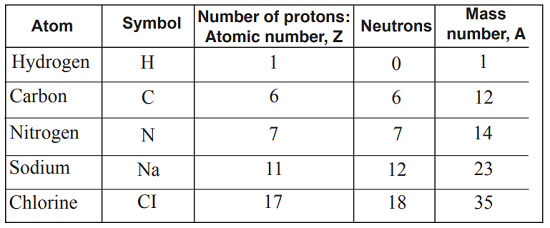Example
Calculate the number of neutrons in a chlorine atom given that the atomic number, Z = 17 and mass number, A = 35

The number of neutrons
= A – Z
= 35 – 17
= 18

Usually, the atomic number, Z, and mass number A, of an atom of an element X can be written alongside the symbol of that element, one as a superscript and the other a subscript as shown below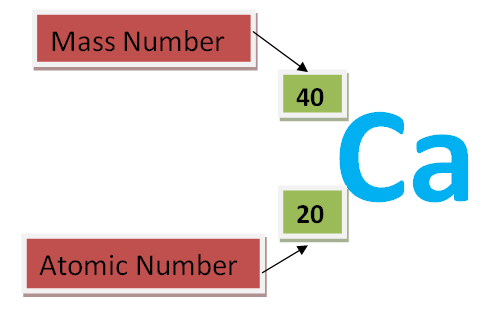NOTE: The top number is referred to as the superscript and bottom number as the subscript.

### Isotopes

Isotopes are atoms of the same element, which have the same number of protons in the nucleus (atomic number) but different mass numbers.
There are two examples below.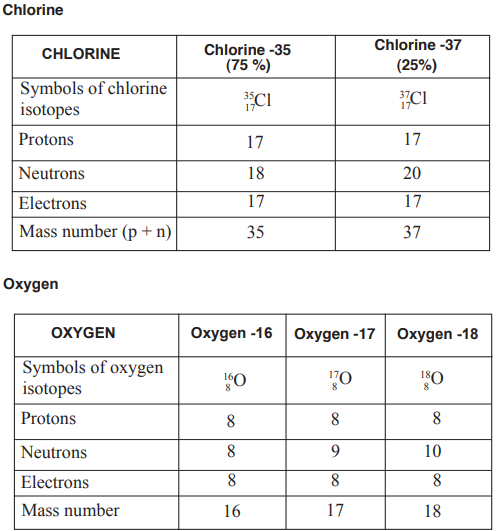### Relative Atomic Mass

Originally, the mass of a hydrogen atom was taken as the standard reference atom. Its atomic mass was arbitrarily fixed as one unit i.e H = 1.

The mass of any other element was found by comparing its mass with that of hydrogen.The idea wasto find out howmany timesthe atomof another element is as heavy as one atom of hydrogen hence relative atomic mass (R.A.M)

Relative Atomic Mass = mass of 1 atom of the element divided by mass of 1 atom of hydrogen

For instance an oxygen atom (O), has a mass of 16. This means one oxygen atom is 16 times heavier than one atom of hydrogen.

NOTE
Relative atomic mass has no units. It is a ratio of two masses. The relative atomic masses are not whole numbers like mass numbers. This is because the abundance of isotopes of an element is different.

Example
Chlorine consists of two isotopes, chlorine – 35 and chlorine – 37 in the ratio 3 : 1. Calculate the relative atomic mass (R.A.M) of chlorine.

Suppose the sample contains 4 atoms of chlorine, in the ratio 3 : 1
3 atoms will each have a mass of 35
1 atom will have a mass of 37
The total mass of 35Cl = 35 × 3
While the total mass of 37Cl = 37 × 1
Therefore, the average mass of chlorine atoms will be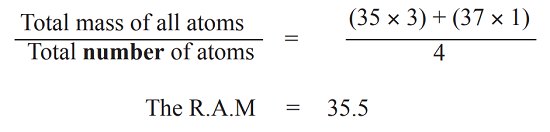## The Period Table

The periodic table is organised as a big grid.
The elements are placed in specific columns because of the way they behave.
They have similar chemical properties because they have the same number of electrons in their outermost energy level.
In a periodic table, each of the rows is called a period.
A column in the periodic table is called a group.
The group number also is the number of electrons in the outermost energy level, and the period is also the number of energy levels in the element.

### Electron Arrangement and the Periodic Table

Given the atomic number of an element, we can write the electron arrangement in shorthand
The atomic numbers of X, Y and Z are 12, 13 and 17 respectively. Write the electron arrangement of each.

X - 2.8.2
Y - 2.8.3
Z - 2.8.7

All these three elements are in period 3 because each has three energy levels. X is in group two, Y in group 3 and Z in group 7, following the number of electrons in their outermost energy levels.

Hydrogen is a unique element. It is placed in Group I as its atoms have one electron in the outermost energy level. It also fits in Group VII because its atoms have one electron short of a fully filled first energy level.

### Ion Formation

Group VIII elements are described as being stable. This means that their outermost energy levels have the maximum number of electrons that they can accommodate.

The process of achieving a stable electron arrangement of 2 or 8 electrons in the outermost energy level is known as duplet e.g. in helium or octet rule respectively. In order to achieve a more stable outermost electron arrangement of 2 or 8 electrons, electrons must be gained or lost in the outermost energy level.

When an atom gains or loses an electron or electrons, the particle formed is charged either negatively (–ve) or positively (+ve). Such a particle is called an ion.

Formation of lithium and magnesium ions
A lithium atom has an electron arrangement of 2.1. It has only one electron in the outermost energy level. For it to be stable, it can either lose one electron to have an electron arrangement of 2 or gain seven electrons to attain an arrangement of 2.8.

More energy will be required for the atom to gain 7 electrons than to lose 1 electron. Therefore, it is easier for a lithium atom to lose 1 electron.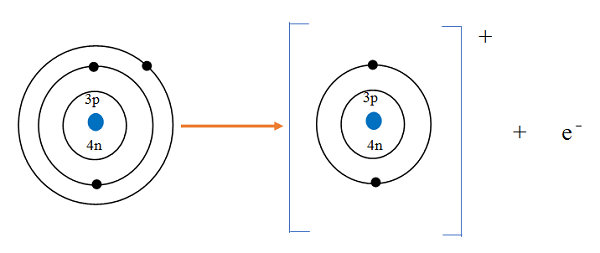Lithium has 3 protons in the nucleus which are positively charged and 4 neutrons which are neutral. The charge in the nucleus is +3. The electrons in the two energy levels are three and are negatively charged. This gives charge of -3 outside the nucleus. The net charge of the atom is +3 – 3 = 0. That is why a lithium atom is neutral.

When a lithium atom loses one electron, the charge of the nucleus remains as +3 whereas outside the nucleus the charge reduces to –2. The net charge then becomes +3 –2 = +1. Lithium ion is then represented as Li+ but not Li+1.

For magnesium, it is easier to lose two electrons than gain six electrons. Therefore a magnesium ion is formed by loss of two electrons.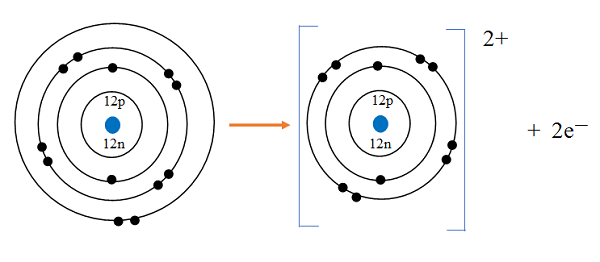Formation of fluoride and sulphide ions
Afluorine atom hasthe electron arrangement of 2.7. For fluorine atom to attain a stable electron arrangement of 2.8, it will either lose seven electrons or gain one electron respectively. Much more energy will be required for fluorine to lose seven electrons than to gain one electron. For this reason it is easier for fluorine to gain one electron than to lose the seven electrons. Therefore, a fluorine atom forms a fluoride ion by gaining one electron.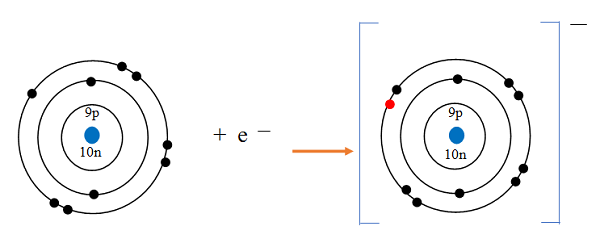The table below shows electron arrangement of some ions.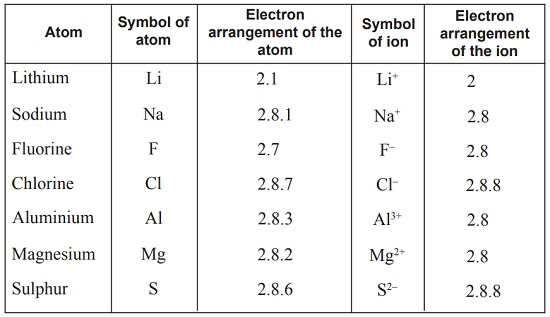### Ionisation Energy

We have seen from the structure of atoms that protons in the nucleus which are positively charged (+ve) attract electrons which are negatively charged (–ve) and located in the energy level.

In order to remove electron, we must overcome this force of attraction. In other words we must supply energy to pull off the electron(s).

The energy supplied is called ionisation energy. The unit for this energy is called joule(J) which can be converted to kilojoules(kJ).

Ionisation energy is defined as the energy required to remove electron(s) from an atom in gaseous state to produce an ion.

### Electron Affinity

When an electron tries to enter the outermost energy level, it will be repelled by the electrons which are already there. So “force” is required to put the electrons into the energy level. This “force” is a form of energy. This energy is known as electron affinity. The ions formed are always negatively charged.

### Oxidation Numbers

The oxidation number of an ion is simply the charge on the ion. + or – sign is written before the number unlike the charge.

Why is the oxidation number of H, +1, yet it is a non-metal? Sometimes a hydrogen atom loses an electron like a metal. During other reactionsit gains an electron to achieve the helium electron arrangement.

### Valencies

The number of electrons an atom requires to attain the stable noble gas electron arrangement is known as valency or combining power of the atom or group of atoms. A group of atoms which occur in compounds but cannot exist on its own is called a radical, for example sulphate, SO42- , hydroxide, OH- , etc.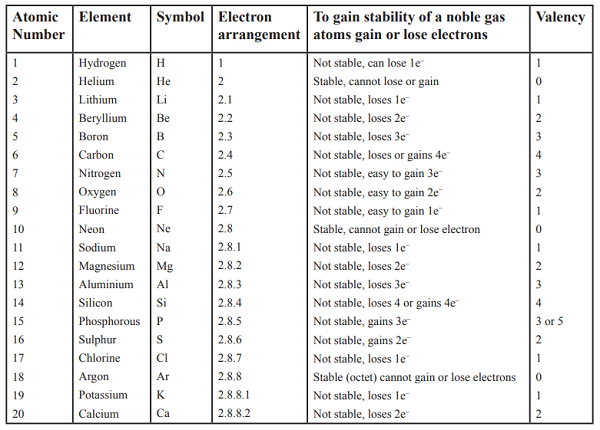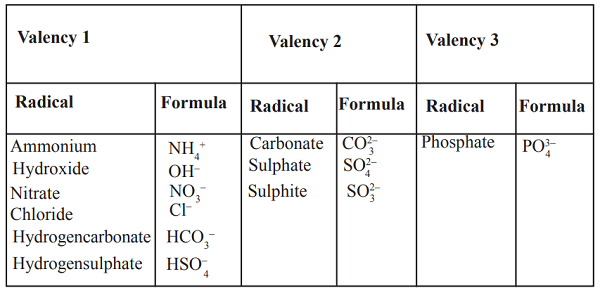### Writing Chemical Formulae

The formula of a compound shows the atoms present in the compound in their simplest ratio. The formula of sodium chloride is NaCl. The formula for water is H2O.

When writing the formula, metals are always written first. For example it is not correct to write ClNa or Br2Ca even though it gives us the same information. The correct way is NaCl and CaBr2 respectively.

### Balancing Chemical Equations

In order for an equation to describe a reaction accurately, the equation must be balanced. Chemical reactions should always follow the law of conservation of mass so that the total mass of reactants must be equal to the total mass of products.

An equation can be balanced using a number of rules. Let us follow these rules to balance the following equation.

Mg + O2 → MgO

Rule number 1
Write the equation using correct formulae for the reactants and products Magnesium + oxygen → Magnesium oxide

Mg + O2 → MgO

Rule number 2
Count the number of atoms of each element in the reactants and in the products.
We notice that the oxygen atoms are not equal. We have 2 atoms on the left and only one on the right.

Rule number 3
To make oxygen atoms equal, balance the equation by writing numbers in front of the formula. Remember that 1 is assumed to be there already. Therefore start by inserting 2. If this does not balance, go to 3, 4 until the equation is balanced. Usually we do not go to very big numbers.

2Mg + O2 → 2MgO

The number 2 now balances the equation. When you have 2O2 it means two oxygen molecules. The number in front of a formula means, everything following is multiplied by that number.

Rule number 4
Count again the number of atoms of each element on the reactants and product sides.

Rule number 5
Insert the correct state symbols for each substance.

2Mg (s) + O2 (g) → 2MgO (s)